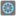• # I can't check "just even" wrong answers well?

## Question related to missionEven the Last

def checkio(array):

```result=0
leng=len(array)
if leng==0:
return 0
for i in array:
if (i%2==0):
result += i

#result=result*array
return result*array[-1]
```

if name == 'main': print('Example:') print(checkio([0, 1, 2, 3, 4, 5]))

```assert checkio([0, 1, 2, 3, 4, 5]) == 30, "(0+2+4)*5=30"
assert checkio([1, 3, 5]) == 30, "(1+5)*5=30"    #  <----------What solution is this? Is this the content of the task?
```8